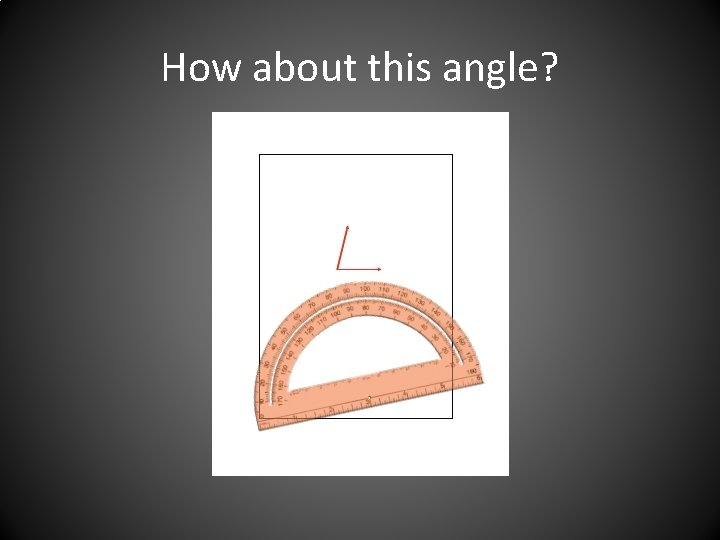# How to Use a Protractor A tool to

• Slides: 16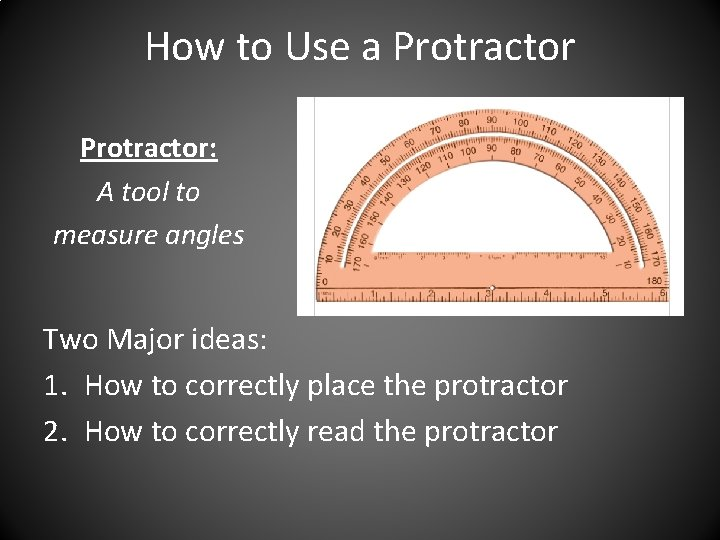How to Use a Protractor: A tool to measure angles Two Major ideas: 1. How to correctly place the protractor 2. How to correctly read the protractor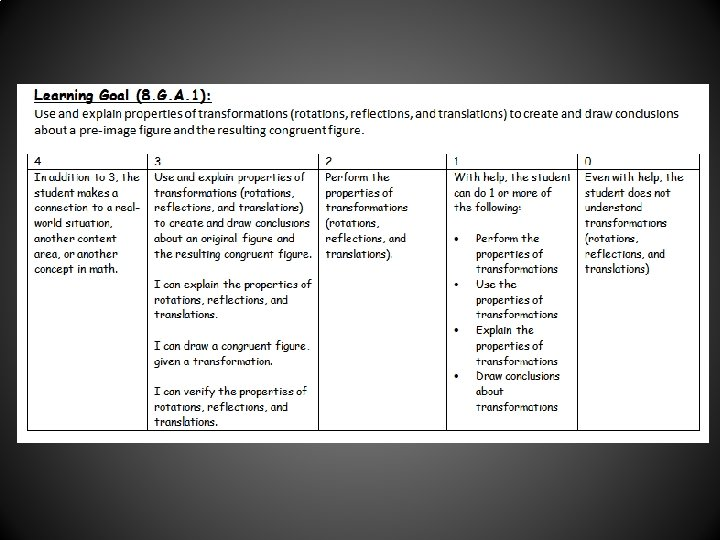Part of the Protractor Outer Scale Inner Scale Zero-Edge Center MarkVocabulary Words Zero Edge Always the same level as the 0 degree mark Inner Scale The numbers on the inner edge of the protractor; the scale goes from right to left. Center Mark Always at the middle of the zero-edge Outer Scale The numbers on the outer edge of the protractor; the scale goes from left to right.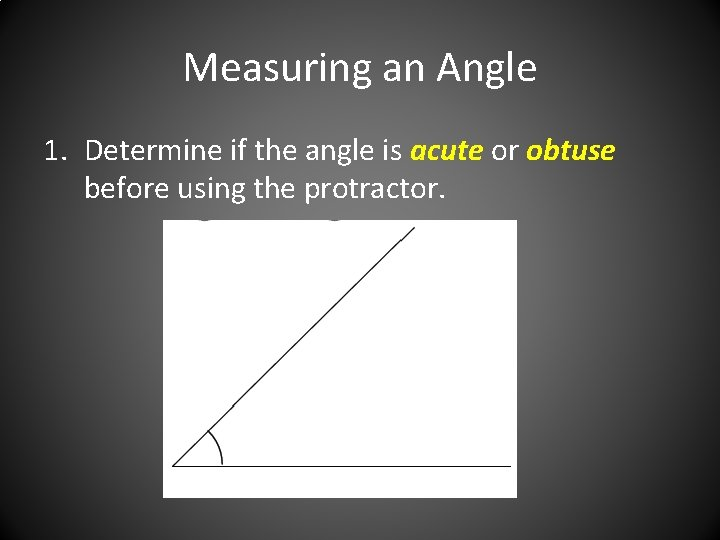Measuring an Angle 1. Determine if the angle is acute or obtuse before using the protractor.Acute, Right or Obtuse? • Right Angle – an angle whose measure is 90˚. • Acute Angle – an angle whose measure is greater than zero but less than 90˚. • Obtuse Angle – an angle whose measure is greater than 90 but less than 180˚.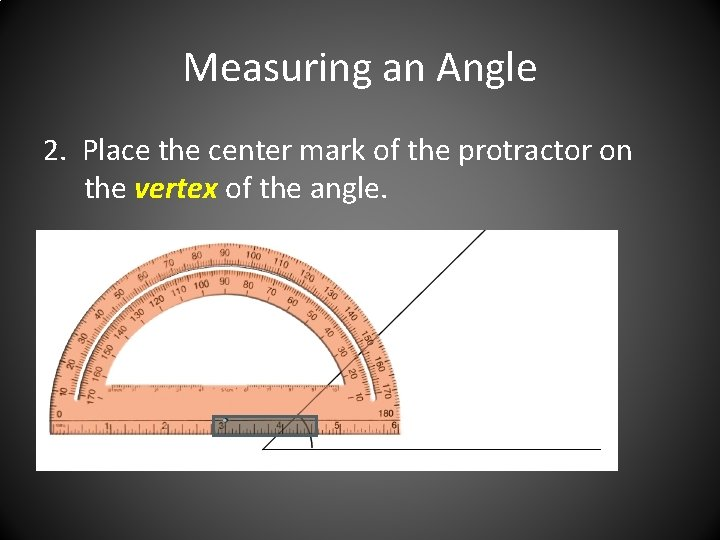Measuring an Angle 2. Place the center mark of the protractor on the vertex of the angle.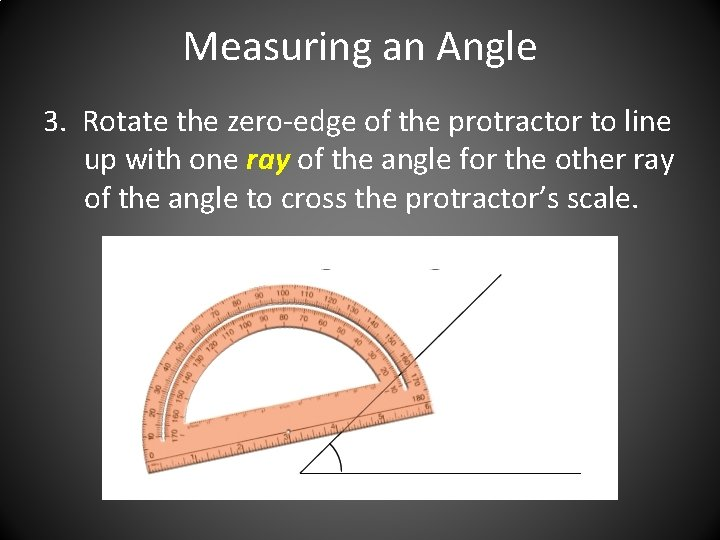Measuring an Angle 3. Rotate the zero-edge of the protractor to line up with one ray of the angle for the other ray of the angle to cross the protractor’s scale.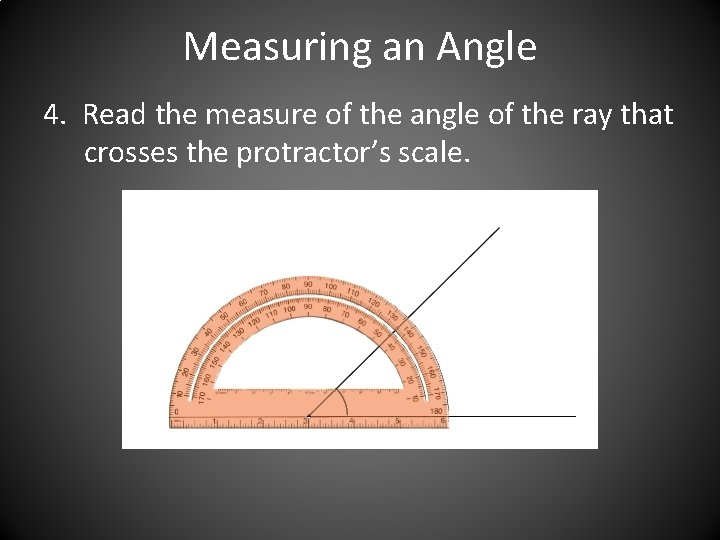Measuring an Angle 4. Read the measure of the angle of the ray that crosses the protractor’s scale.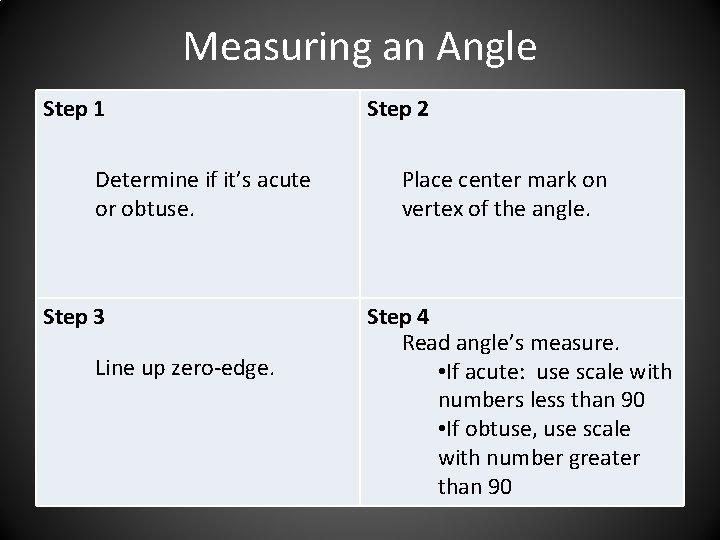Measuring an Angle Step 1 Determine if it’s acute or obtuse. Step 3 Line up zero-edge. Step 2 Place center mark on vertex of the angle. Step 4 Read angle’s measure. • If acute: use scale with numbers less than 90 • If obtuse, use scale with number greater than 90Common Mistakes 1. Using the incorrect scale. 2. The center mark is not on the vertex. – This usually happens after rotating the protractor. Always check your centering when moving the protractor. 3. Counting the marks in the wrong direction. – Pay attention to whether the numbers are increasing or decreasing.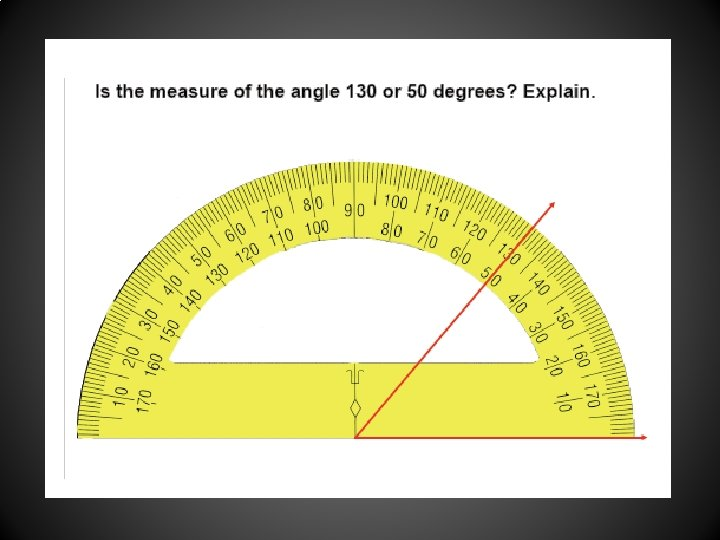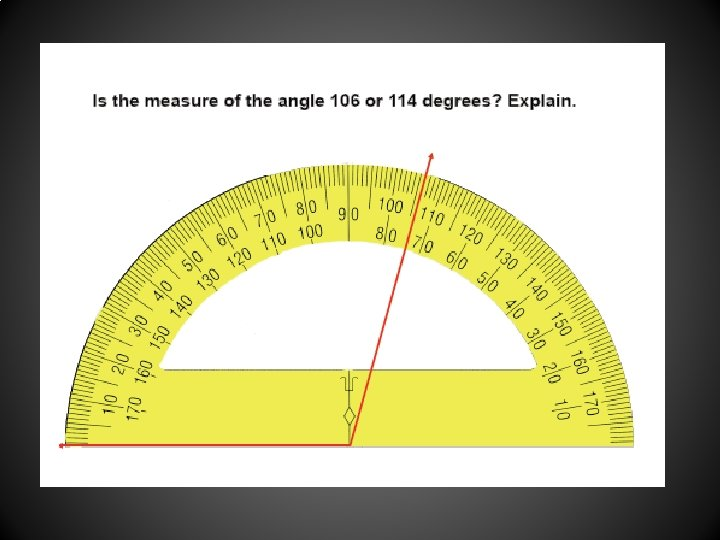How would you measure these angles?